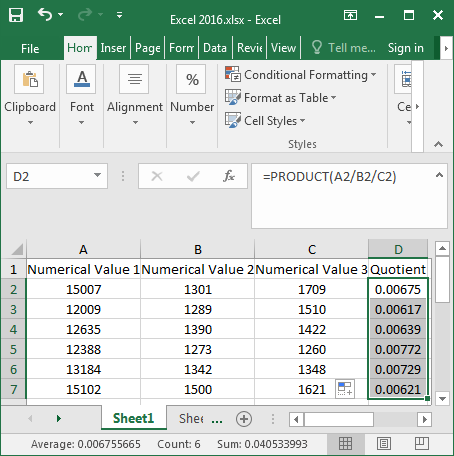• Blog>
• Office>
• How to Calculate Quotient of the Cells in Excel 2016

# How to Calculate Quotient of the Cells in Excel 2016There are not only powerful formulas in Excel, but also enormously strong computational functions. It can cover almost every aspect of life that needs to be calculated. Excel can be used to calculate addition, subtraction, multiplication, and division as well as average value. Now I’m going to teach you calculate a quotient formula for Excel 2016, which is very practical and convenient. It can be used flexibly later!

## Steps to calculate quotient of the cells in Excel 2016

There are a lot of functions in Excel. It can facilitate your work and save you a lot of time and energy. Now let’s see how the division function is used in Excel 2016.

Step 1: Open the target Excel 2016.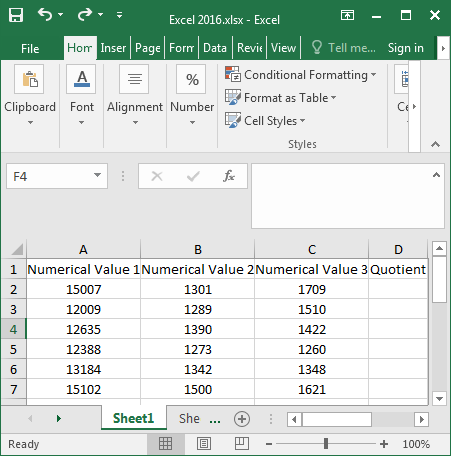Step 2: Type the division formula “=A2/B2/C2” in the D2 cell and press Enter.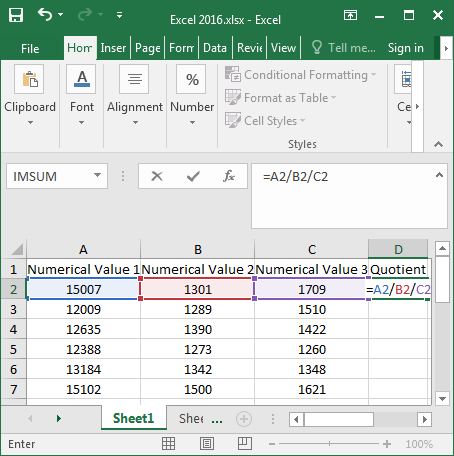Or enter the formula “=PRODUCT(=A2/B2/C2)” in the D2 cell and press Enter to display the quotient in it.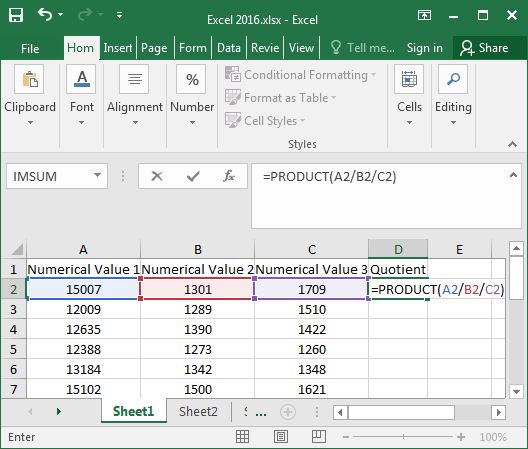Step 3: Place the mouse on the lower right corner of the D2 cell, and a bold black “+” symbol appears. Hold down the “+” with the left mouse button and drag it all the way down.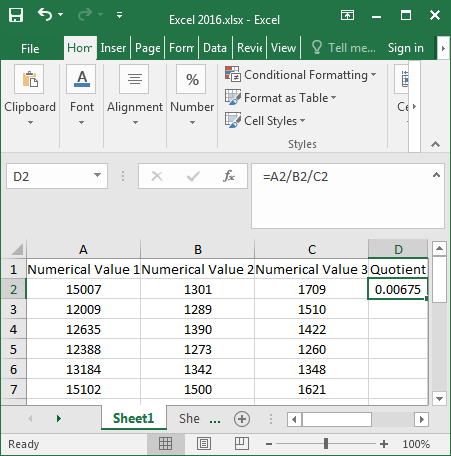Step 4: At this time, as you can see, all of the division results for column D2 have been figured out below.